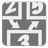Digit Stack
Elementary+
English FR JA RU

In computer science, a stack is a particular kind of data type or collection in which the principal operations in the collection are the addition of an entity to the collection (also known as push) and the removal of an entity (also known as pop). The relation between the push and pop operations is such that the stack is a Last-In-First-Out (LIFO) data structure. In a LIFO data structure, the last element added to the structure must be the first one to be removed. Often a peek, or top operation is also implemented, returning the value of the top element without removing it.

We will emulate the stack process with Python. You are given a sequence of commands:
- "PUSH X" -- add X in the stack, where X is a digit.
- "POP" -- look and remove the top position. If the stack is empty, then it returns 0 (zero) and does nothing.
- "PEEK" -- look at the top position. If the stack is empty, then it returns 0 (zero).
The stack can only contain digits.

You should process all commands and sum all digits which were taken from the stack ("PEEK" or "POP"). Initial value of the sum is 0 (zero).

Let's look at an example, here’s the sequence of commands:
["PUSH 3", "POP", "POP", "PUSH 4", "PEEK", "PUSH 9", "PUSH 0", "PEEK", "POP", "PUSH 1", "PEEK"]

Command Stack Sum
PUSH 3 3 0
POP 0+3
POP 3+0
PUSH 4 4 3
PEEK 4 3+4
PUSH 9 4,9 7
PUSH 0 4,9,0 7
PEEK 4,9,0 7+0
POP 4,9 7+0
PUSH 1 4,9,1 7
PEEK 4,9,1 7+1=8

Input: A sequence of commands as a list of strings.

Output: The sum of the taken digits as an integer.

Example:

```digit_stack(["PUSH 3", "POP", "POP", "PUSH 4", "PEEK", "PUSH 9", "PUSH 0", "PEEK", "POP", "PUSH 1", "PEEK"]) == 8
digit_stack(["POP", "POP"]) == 0
digit_stack(["PUSH 9", "PUSH 9", "POP"]) == 9
digit_stack([]) == 0
```

How it is used: Stacks have numerous applications. We see stacks in everyday life, from the books in our library, to the blank sheets of paper in our printer tray. All of them follow the Last In First Out (LIFO) logic, that is when we add a book to a pile of books, we add it to the top of the pile, whereas when we remove a book from the pile, we generally remove it from the top of the pile.

Precondition:
0 ≤ len(commands) ≤ 20;
all(re.match("\APUSH \d\Z", c) or с == "POP" or c == "PEEK" for c in commands)